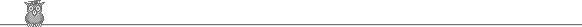### 27th USAMO 1998Problem B3

What is the largest number of the quadrilaterals formed by four adjacent vertices of an convex n-gon that can have an inscribed circle?

Solution

Take a regular n-gon and slice off alternate corners (until [n/2] corners have been cut). Specifically, if the vertices are A1, A2, ... , An, then we slice off the corners at A1, A3, A5, ... Am, where m = n-1 if n is even, or n-2 if n is odd. At each corner Ai which we slice, we take the inscribed circle to the triangle Ai-1AiAi+1, draw a tangent to it parallel to Ai-1Ai+1 and cut along the tangent. This procedure shows that [n/2] can be achieved.

To show that it is optimal it is sufficient to show that if A, B, C, D, E are adjacent vertices of any polygon and ABCD has an inscribed polygon, then BCDE does not. Since ABCD has an inscribed polygon, AD + BC = AB + CD (if the inscribed circle touches at X on AB and Y on AD, then AX = AY. That and the three similar equations give the result). So if BCDE also has an inscribed polygon, then BE + CD = BC + DE. Hence (adding) AD + BE = AB + DE. But the diagonals AD and BE must meet at some point X. Then AD + BE = AX + XD + BX + XE = (AX + XB) + (DX + XE) > AB + DE. Contradiction.